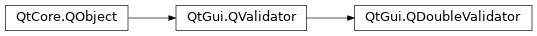# QDoubleValidator¶

The `QDoubleValidator` class provides range checking of floating-point numbers. More## Detailed Description¶

`QDoubleValidator` provides an upper bound, a lower bound, and a limit on the number of digits after the decimal point. It does not provide a `fixup()` function.

You can set the acceptable range in one call with `setRange()` , or with `setBottom()` and `setTop()` . Set the number of decimal places with `setDecimals()` . The `validate()` function returns the validation state.

`QDoubleValidator` uses its `locale()` to interpret the number. For example, in the German locale, “1,234” will be accepted as the fractional number 1.234. In Arabic locales, `QDoubleValidator` will accept Arabic digits.

Note

The `NumberOptions` set on the `locale()` also affect the way the number is interpreted. For example, since `RejectGroupSeparator` is not set by default, the validator will accept group separators. It is thus recommended to use `toDouble()` to obtain the numeric value.

class PySide2.QtGui.QDoubleValidator([parent=None])

PySide2.QtGui.QDoubleValidator(bottom, top, decimals[, parent=None])

param parent:

`PySide2.QtCore.QObject`

param bottom:

`double`

param top:

`double`

param decimals:

int

Constructs a validator object with a `parent` object that accepts any double.

Constructs a validator object with a `parent` object. This validator will accept doubles from `bottom` to `top` inclusive, with up to `decimals` digits after the decimal point.

PySide2.QtGui.QDoubleValidator.Notation

This enum defines the allowed notations for entering a double.

Constant

Description

QDoubleValidator.StandardNotation

The string is written as a standard number (i.e. 0.015).

QDoubleValidator.ScientificNotation

The string is written in scientific form. It may have an exponent part(i.e. 1.5E-2).

PySide2.QtGui.QDoubleValidator.bottom()
Return type:

`double`

This property holds the validator’s minimum acceptable value.

By default, this property contains a value of -infinity.

PySide2.QtGui.QDoubleValidator.bottomChanged(bottom)
Parameters:

bottom`double`

PySide2.QtGui.QDoubleValidator.decimals()
Return type:

int

This property holds the validator’s maximum number of digits after the decimal point.

By default, this property contains a value of 1000.

PySide2.QtGui.QDoubleValidator.decimalsChanged(decimals)
Parameters:

decimals – int

PySide2.QtGui.QDoubleValidator.notation()
Return type:

`Notation`

This property holds the notation of how a string can describe a number.

By default, this property is set to `ScientificNotation` .

`Notation`

PySide2.QtGui.QDoubleValidator.notationChanged(notation)
Parameters:

notation`Notation`

PySide2.QtGui.QDoubleValidator.setBottom(arg__1)
Parameters:

arg__1`double`

This property holds the validator’s minimum acceptable value.

By default, this property contains a value of -infinity.

PySide2.QtGui.QDoubleValidator.setDecimals(arg__1)
Parameters:

arg__1 – int

This property holds the validator’s maximum number of digits after the decimal point.

By default, this property contains a value of 1000.

PySide2.QtGui.QDoubleValidator.setNotation(arg__1)
Parameters:

arg__1`Notation`

This property holds the notation of how a string can describe a number.

By default, this property is set to `ScientificNotation` .

`Notation`

PySide2.QtGui.QDoubleValidator.setRange(bottom, top[, decimals=0])
Parameters:
• bottom`double`

• top`double`

• decimals – int

Sets the validator to accept doubles from `minimum` to `maximum` inclusive, with at most `decimals` digits after the decimal point.

PySide2.QtGui.QDoubleValidator.setTop(arg__1)
Parameters:

arg__1`double`

This property holds the validator’s maximum acceptable value.

By default, this property contains a value of infinity.

PySide2.QtGui.QDoubleValidator.top()
Return type:

`double`

This property holds the validator’s maximum acceptable value.

By default, this property contains a value of infinity.

PySide2.QtGui.QDoubleValidator.topChanged(top)
Parameters:

top`double`